# Imaginary and Complex Numbers: Tutoring Solution Chapter Exam

Exam Instructions:

Choose your answers to the questions and click 'Next' to see the next set of questions. You can skip questions if you would like and come back to them later with the yellow "Go To First Skipped Question" button. When you have completed the practice exam, a green submit button will appear. Click it to see your results. Good luck!

Answered 0 of 25

### Page 1

#### Question 1 1. Simplify.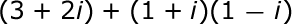#### Question 3 3. What would be the first step in dividing the complex numbers below?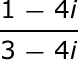#### Question 4 4. Simplify.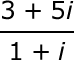#### Question 5 5. What type of solution(s) would you get from the equation below?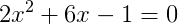### Page 2

#### Question 8 8. Find the roots of the equation below: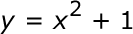#### Question 9 9. Simplify.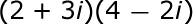#### Question 10 10. Simplify.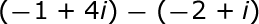### Page 3

#### Question 13 13. Which number is represented by the graph below?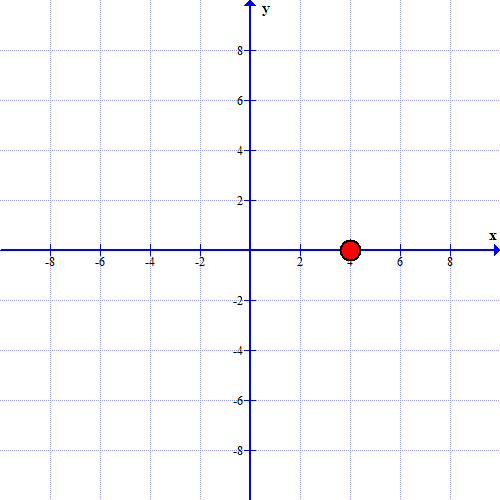#### Question 14 14. What is the simplified value of the expression below?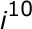#### Question 15 15. Simplify.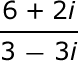### Page 4

#### Question 16 16. What is the complex conjugate of the expression below?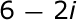#### Question 17 17. How many roots does the equation below have?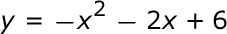#### Question 18 18. Which statement MUST be true about the equation below?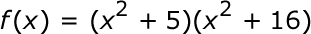#### Question 19 19. What is the most simplified form of the expression below?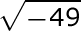### Page 5

#### Question 23 23. Solve the equation below: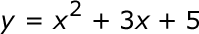#### Question 24 24. Simplify.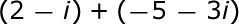#### Question 25 25. Which number is represented by the graph below?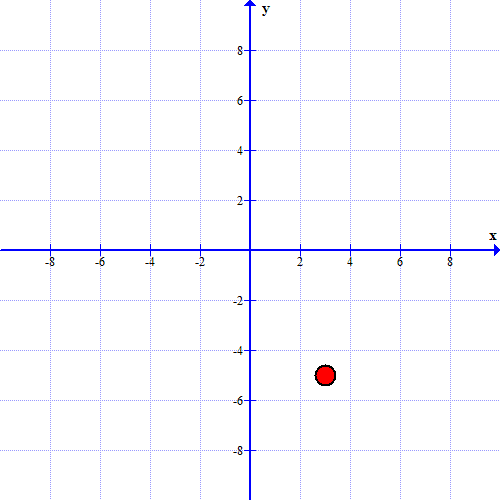#### Imaginary and Complex Numbers: Tutoring Solution Chapter Exam Instructions

Choose your answers to the questions and click 'Next' to see the next set of questions. You can skip questions if you would like and come back to them later with the yellow "Go To First Skipped Question" button. When you have completed the practice exam, a green submit button will appear. Click it to see your results. Good luck!

Support